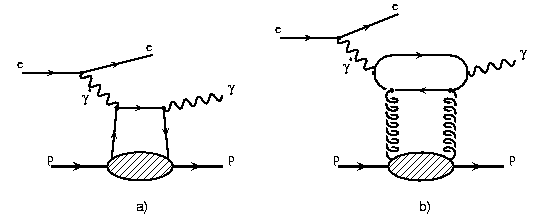# Electron-Proton Scattering

One of the main missions of the electron proton collider HERA is the investigation of the structure of the proton. It is known that the proton consists of quarks and gluons, the so called partons. In electron proton collisions the incoming electron emits a photon which interacts with a parton from the proton. One of the main results achieved was the precise measurement of the so called parton density functions  fi(x,Q2) which denotes the probability to find a parton of type i with a certain momentum fraction x at a resolution scale Q where 1/Q is the wavelength of the incoming photon which probes the proton. At HERA a large range in Q2 is available which enables us to study the proton structure at large and small distances.

# Deeply Virtual Compton Scattering (DVCS)

In a certain class of interactions, the so called diffractive events, the photon interacts with more than one parton of the incoming proton. This kind of events have already been observed in hadron-hadron colliders three decades ago and nowadays it can be understood on the basis of modern theories in terms of quarks and gluons. The large number of this kind of events (~10%) was unexpected when HERA was switched on and initiated a huge research activity.

The simplest model of diffraction which is the exchange of two partons can be tested by studying the DVCS process which is shown in the following figure.

###In diagram a) a photon is emitted by the incoming electron which then interacts with a quark originating from the incoming proton. The quark emits a photon and enters the proton again. Thus the proton has the opportunity to stay intact. In diagram b) the incoming photon interacts indirectly via a quark loop with two gluons of the incoming proton.  The real photon is also emitted from the quark line.
The main goal of studying the DVCS process is to investigate the corellations between the two exchanged partons measuring the so called skewed parton distributions which are generalisations of the parton distributions. Presently the knowledge of this distributions is almost zero apart from some theoretical constraints.
Here thefirst measurement for the so far unknown cross section of the DVCS process is presented.

# The Measurement

In both reactions the signature consists of an electron, a photon and a proton. In the following figure an event is shown which was collected by the H1 detector. The photon is identified by an electromagnetic cluster in the calorimeter in the central part of the detector. The tracking detectors between the interaction point and the calorimeter show no signal of a charged particle. The electron is recognised by a cluster in the electromagnetic calorimeter in the backward region(right) of the H1 detector. The proton is not measured. It leaves the detector through the beam pipe. All other parts of the detector show no signal.

# Background

The main task of this analysis is to control the background, i.e. events originating from other processes which show the same signature in the detector as DVCS events. At HERA another process is present which has the same final state as DVCS. It is the so called Bethe-Heitler(BH) process. A diagram of the BH process is given below.  Due to its apparent simplicity the probability for BH process is precisely known. An excess of events over the BH prediction can thus be attributed to the DVCS process.

# Results

In the diagram below we show one of the results of this measurement. The differential ep cross section (roughly speaking: "The probability to find such events")  as a function of W which is the energy in the photon proton center of mass system. The points show the results of the measurement, the assigned error bars are due to the statistical precision and due to systematic uncertainties. This process is very rare, therefore the statistical uncertainty is large. In total we have observed 172 events of this type. The systematic uncertainty is due to our limited knowledge of the detector on certain physics processes. The blue histogram shows the contribution which is predicted for the Bethe-Heitler process. The measured distribution differs strongly from the predicted cross section for the BH process. This difference is attributed to the DVCS process.

###In order to extract the DVCS cross section the prediction for the BH process has been subtracted which is well known.  In the figure below the cross section for the DVCS cross section is shown  as a function of the kinematic variable Q2. The data(black points) are compared to theoretical predictions. In yellow the prediction by Frankfurt, Freund and Strikman is shown, in blue the prediction by Donnachie and Dosch. Both calculations exhibit a normalisation uncertainty which is indicated as a band. It can be seen that within this normalisation uncertainty the theories describe the measurement.